Скачать презентацию Lecture 10 Clustering 1 Preview n Introduction

• Количество слайдов: 45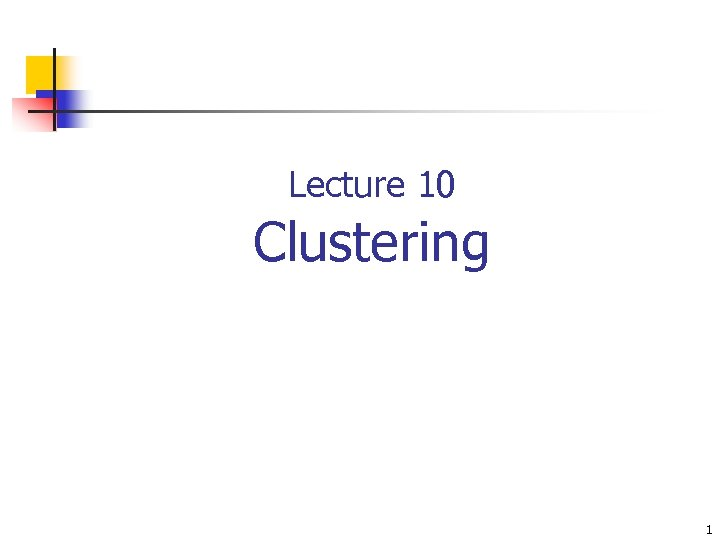Lecture 10 Clustering 1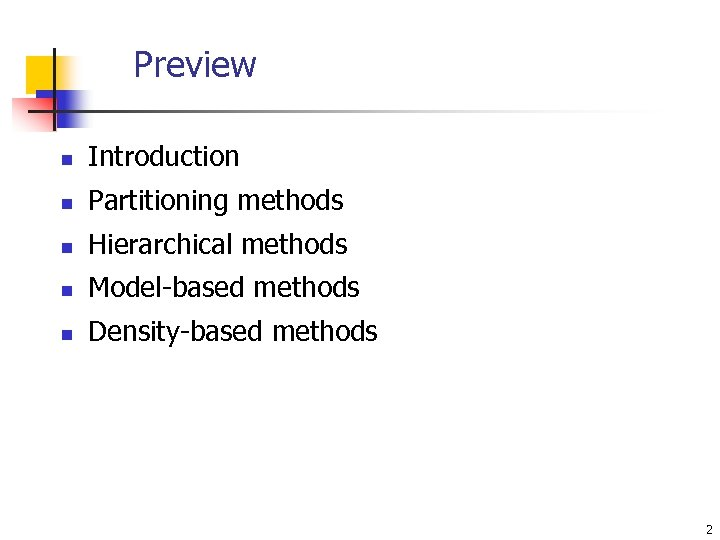Preview n Introduction n Partitioning methods n Hierarchical methods n Model-based methods n Density-based methods 2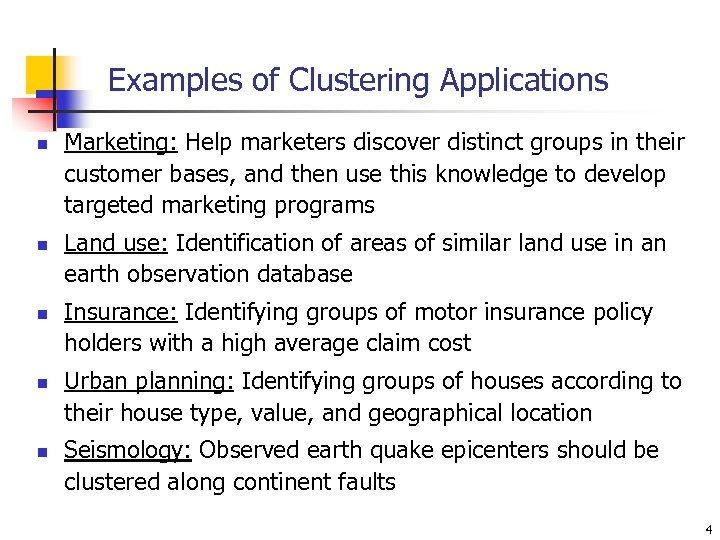Examples of Clustering Applications n n n Marketing: Help marketers discover distinct groups in their customer bases, and then use this knowledge to develop targeted marketing programs Land use: Identification of areas of similar land use in an earth observation database Insurance: Identifying groups of motor insurance policy holders with a high average claim cost Urban planning: Identifying groups of houses according to their house type, value, and geographical location Seismology: Observed earth quake epicenters should be clustered along continent faults 4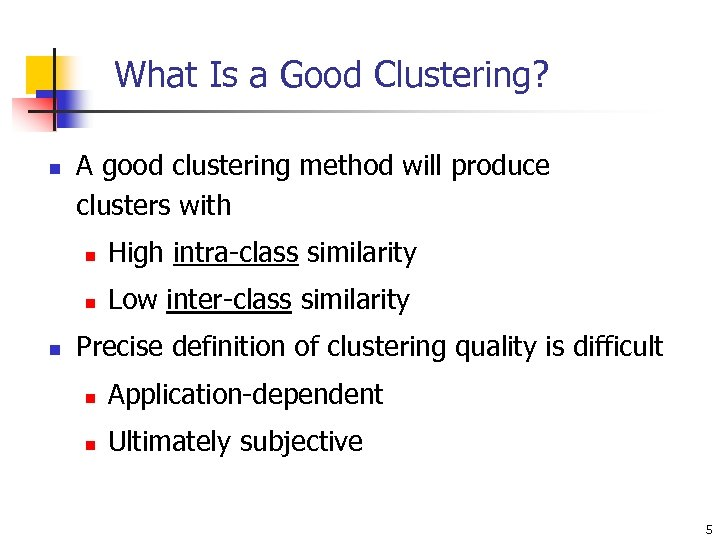What Is a Good Clustering? n A good clustering method will produce clusters with n n n High intra-class similarity Low inter-class similarity Precise definition of clustering quality is difficult n Application-dependent n Ultimately subjective 5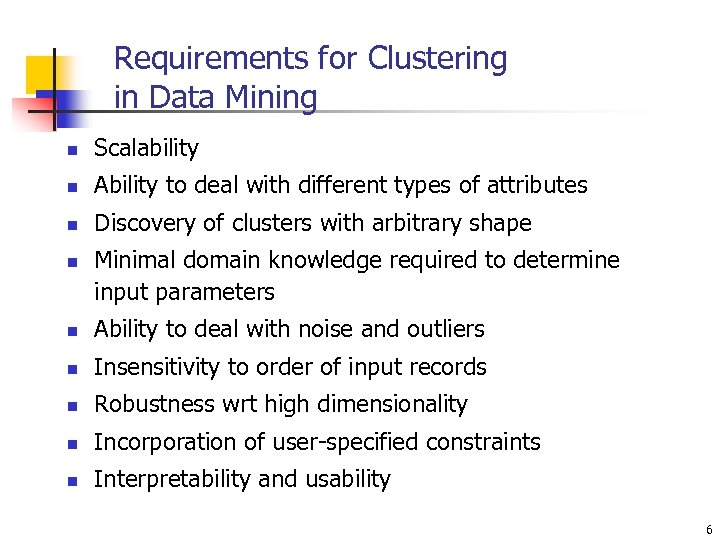Requirements for Clustering in Data Mining n Scalability n Ability to deal with different types of attributes n Discovery of clusters with arbitrary shape n Minimal domain knowledge required to determine input parameters n Ability to deal with noise and outliers n Insensitivity to order of input records n Robustness wrt high dimensionality n Incorporation of user-specified constraints n Interpretability and usability 6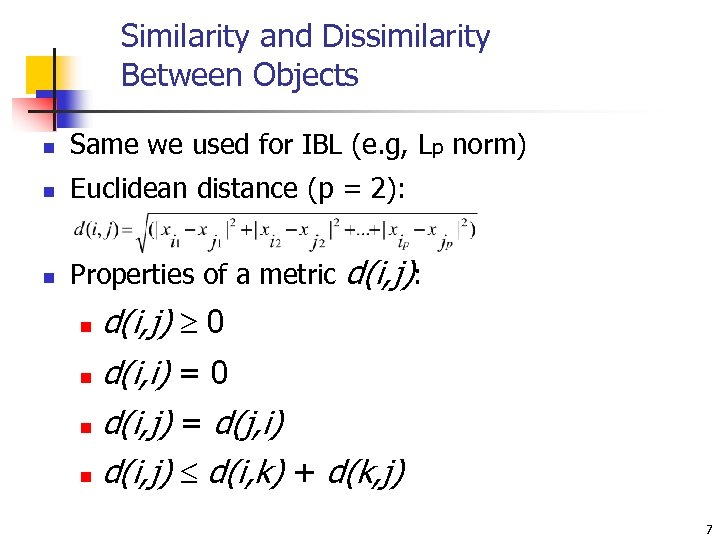Similarity and Dissimilarity Between Objects n Same we used for IBL (e. g, Lp norm) n Euclidean distance (p = 2): n Properties of a metric d(i, j): d(i, j) 0 n d(i, i) = 0 n d(i, j) = d(j, i) n d(i, j) d(i, k) + d(k, j) n 7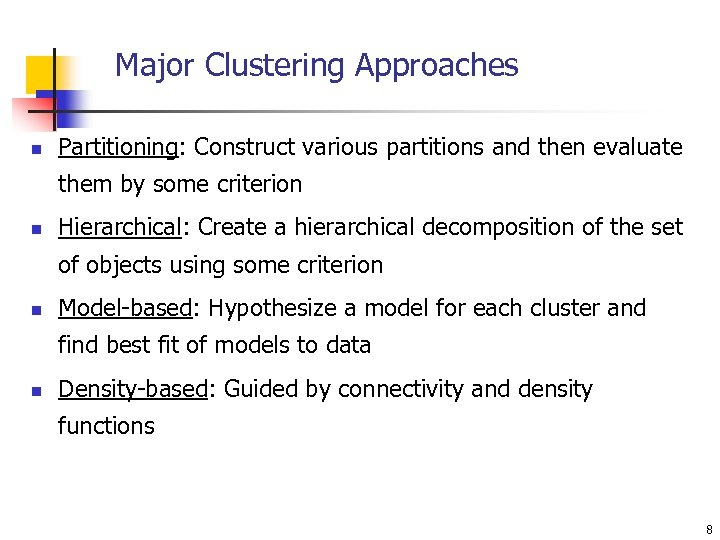Major Clustering Approaches n Partitioning: Construct various partitions and then evaluate them by some criterion n Hierarchical: Create a hierarchical decomposition of the set of objects using some criterion n Model-based: Hypothesize a model for each cluster and find best fit of models to data n Density-based: Guided by connectivity and density functions 8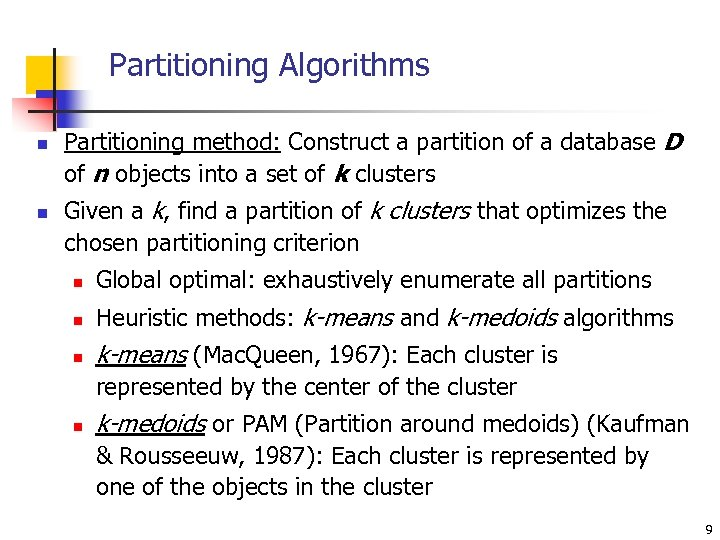Partitioning Algorithms n n Partitioning method: Construct a partition of a database D of n objects into a set of k clusters Given a k, find a partition of k clusters that optimizes the chosen partitioning criterion n Global optimal: exhaustively enumerate all partitions n Heuristic methods: k-means and k-medoids algorithms n k-means (Mac. Queen, 1967): Each cluster is represented by the center of the cluster n k-medoids or PAM (Partition around medoids) (Kaufman & Rousseeuw, 1987): Each cluster is represented by one of the objects in the cluster 9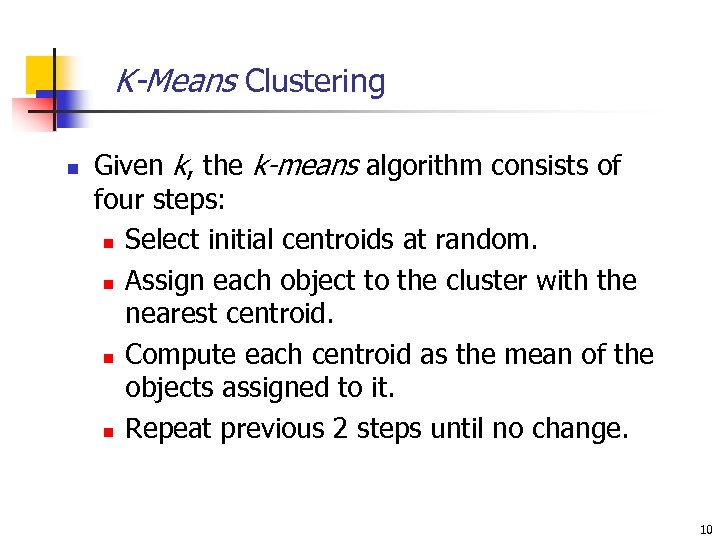K-Means Clustering n Given k, the k-means algorithm consists of four steps: n Select initial centroids at random. n Assign each object to the cluster with the nearest centroid. n Compute each centroid as the mean of the objects assigned to it. n Repeat previous 2 steps until no change. 10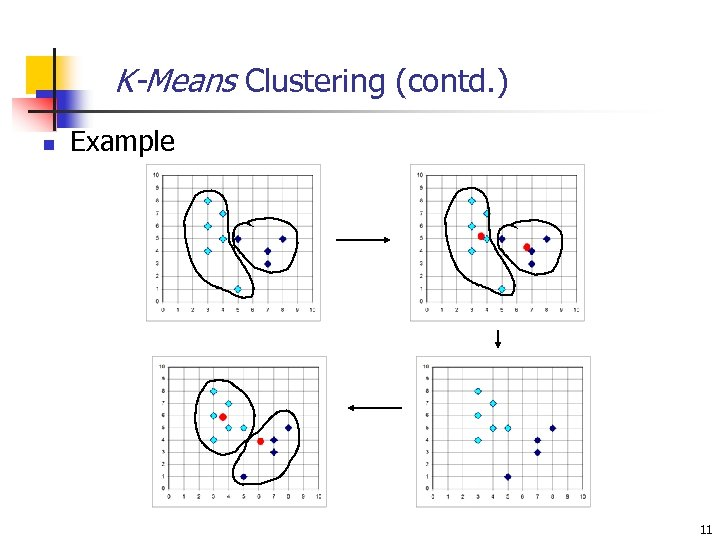K-Means Clustering (contd. ) n Example 11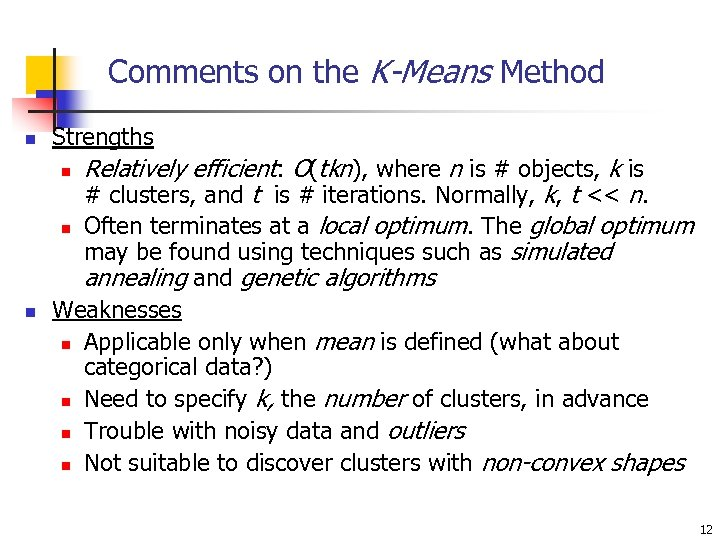Comments on the K-Means Method n Strengths n n n Relatively efficient: O(tkn), where n is # objects, k is # clusters, and t is # iterations. Normally, k, t << n. Often terminates at a local optimum. The global optimum may be found using techniques such as simulated annealing and genetic algorithms Weaknesses n Applicable only when mean is defined (what about categorical data? ) n Need to specify k, the number of clusters, in advance n Trouble with noisy data and outliers n Not suitable to discover clusters with non-convex shapes 12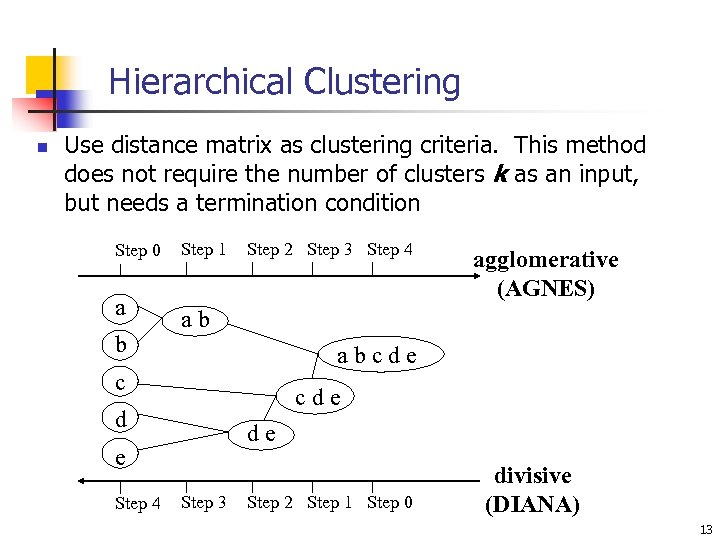Hierarchical Clustering n Use distance matrix as clustering criteria. This method does not require the number of clusters k as an input, but needs a termination condition Step 0 a b Step 1 Step 2 Step 3 Step 4 ab abcde c cde d de e Step 4 agglomerative (AGNES) Step 3 Step 2 Step 1 Step 0 divisive (DIANA) 13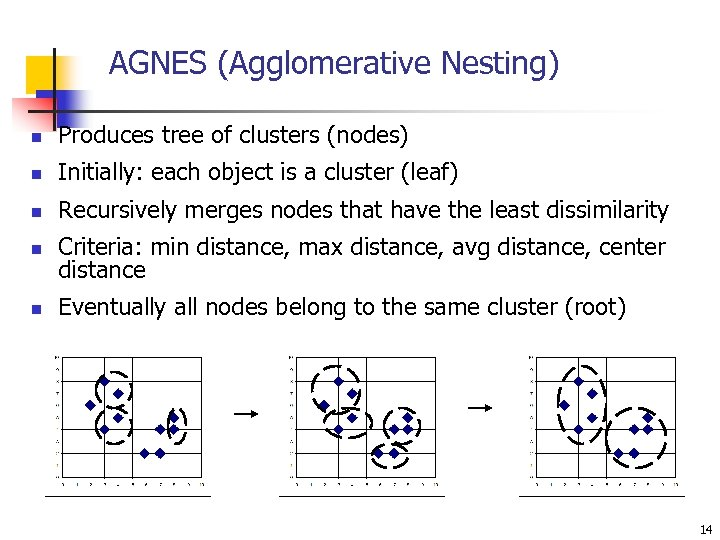AGNES (Agglomerative Nesting) n Produces tree of clusters (nodes) n Initially: each object is a cluster (leaf) n Recursively merges nodes that have the least dissimilarity n Criteria: min distance, max distance, avg distance, center distance n Eventually all nodes belong to the same cluster (root) 14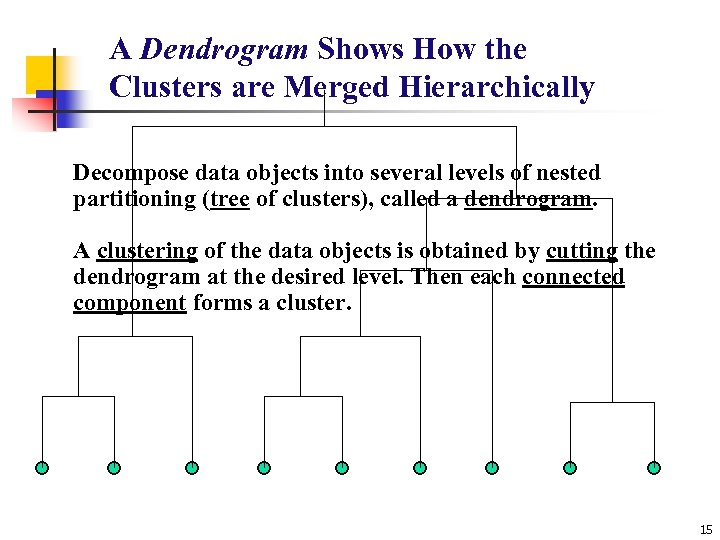A Dendrogram Shows How the Clusters are Merged Hierarchically Decompose data objects into several levels of nested partitioning (tree of clusters), called a dendrogram. A clustering of the data objects is obtained by cutting the dendrogram at the desired level. Then each connected component forms a cluster. 15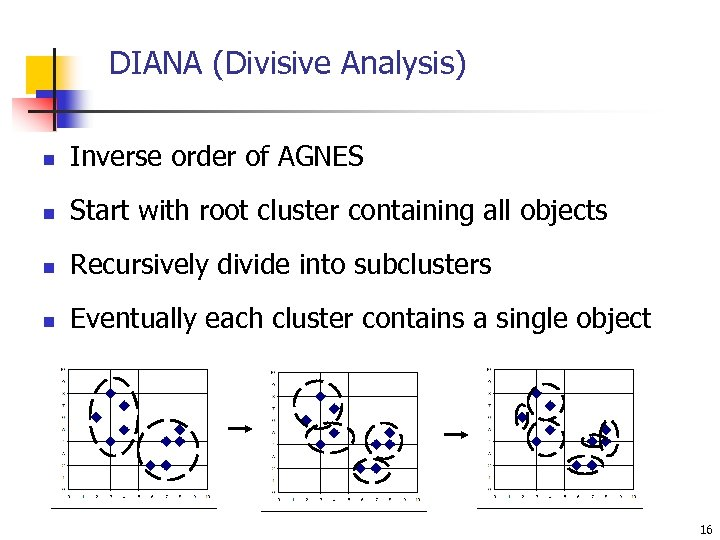DIANA (Divisive Analysis) n Inverse order of AGNES n Start with root cluster containing all objects n Recursively divide into subclusters n Eventually each cluster contains a single object 16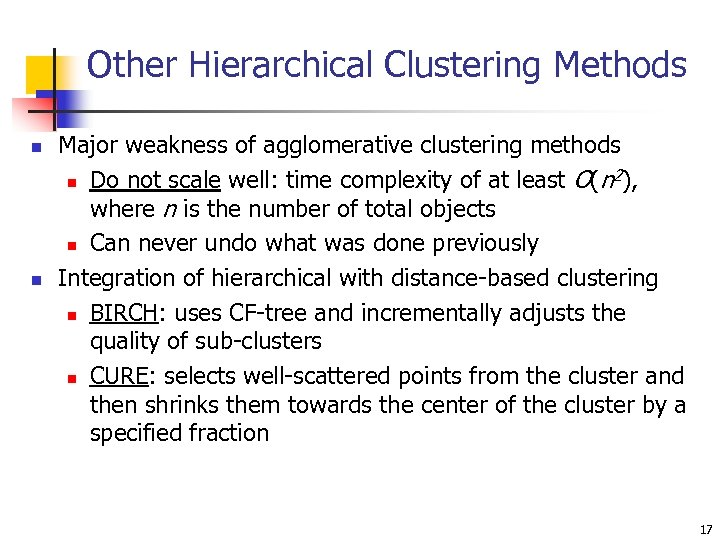Other Hierarchical Clustering Methods n n Major weakness of agglomerative clustering methods 2 n Do not scale well: time complexity of at least O(n ), where n is the number of total objects n Can never undo what was done previously Integration of hierarchical with distance-based clustering n BIRCH: uses CF-tree and incrementally adjusts the quality of sub-clusters n CURE: selects well-scattered points from the cluster and then shrinks them towards the center of the cluster by a specified fraction 17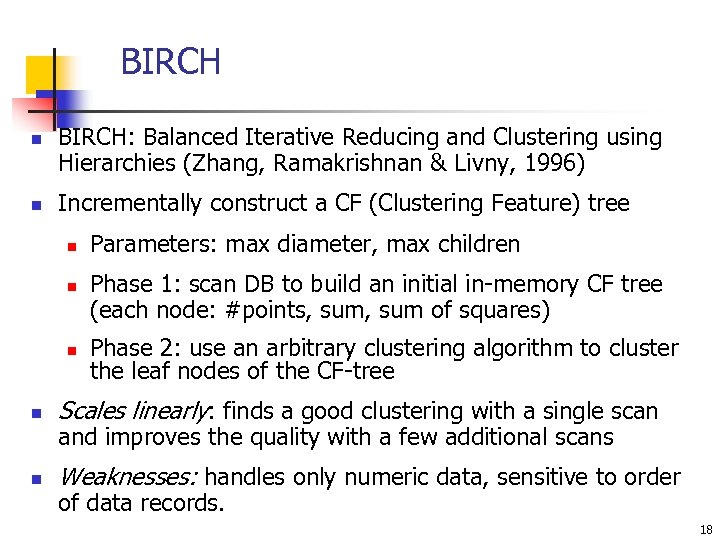BIRCH n n BIRCH: Balanced Iterative Reducing and Clustering using Hierarchies (Zhang, Ramakrishnan & Livny, 1996) Incrementally construct a CF (Clustering Feature) tree n n n Parameters: max diameter, max children Phase 1: scan DB to build an initial in-memory CF tree (each node: #points, sum of squares) Phase 2: use an arbitrary clustering algorithm to cluster the leaf nodes of the CF-tree n Scales linearly: finds a good clustering with a single scan n Weaknesses: handles only numeric data, sensitive to order and improves the quality with a few additional scans of data records. 18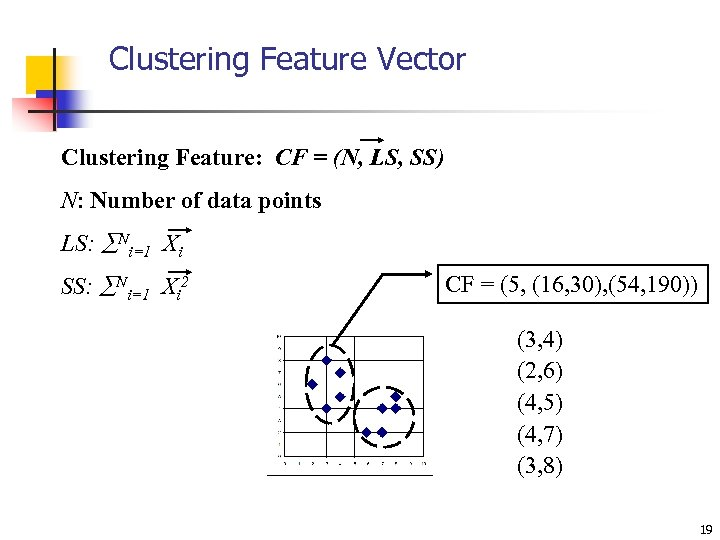Clustering Feature Vector Clustering Feature: CF = (N, LS, SS) N: Number of data points LS: Ni=1 Xi SS: Ni=1 Xi 2 CF = (5, (16, 30), (54, 190)) (3, 4) (2, 6) (4, 5) (4, 7) (3, 8) 19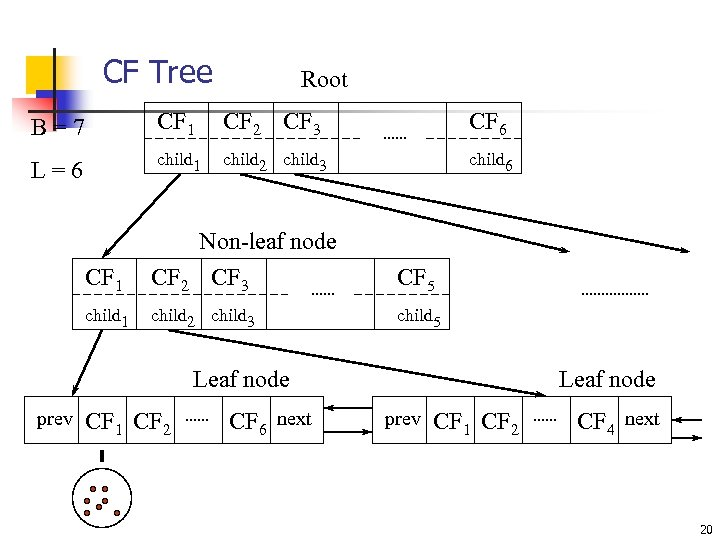CF Tree Root B=7 CF 1 CF 2 CF 3 CF 6 L=6 child 1 child 2 child 3 child 6 CF 1 Non-leaf node CF 2 CF 3 CF 5 child 1 child 2 child 3 child 5 Leaf node prev CF 1 CF 2 CF 6 next Leaf node prev CF 1 CF 2 CF 4 next 20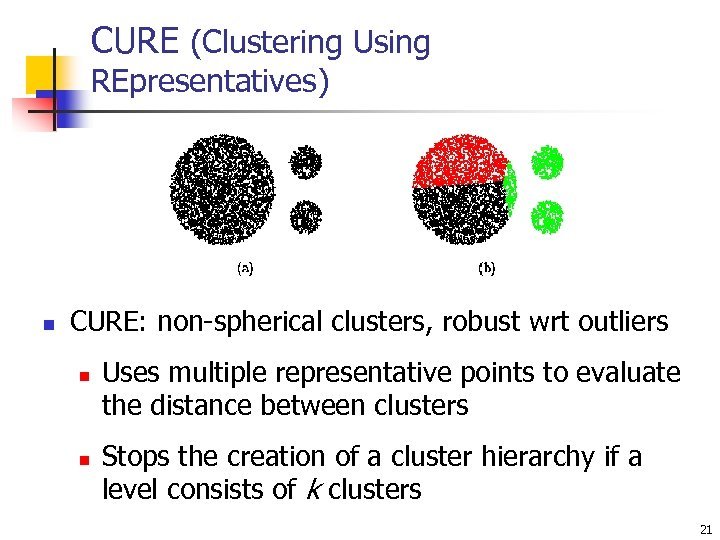CURE (Clustering Using REpresentatives) n CURE: non-spherical clusters, robust wrt outliers n n Uses multiple representative points to evaluate the distance between clusters Stops the creation of a cluster hierarchy if a level consists of k clusters 21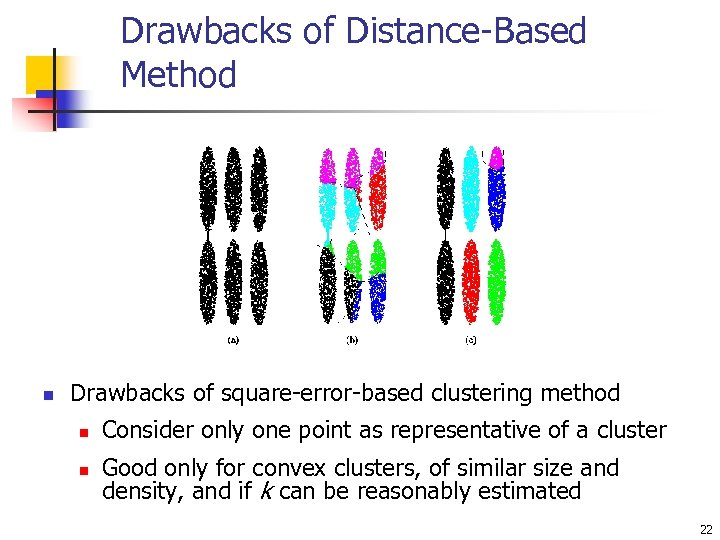Drawbacks of Distance-Based Method n Drawbacks of square-error-based clustering method n n Consider only one point as representative of a cluster Good only for convex clusters, of similar size and density, and if k can be reasonably estimated 22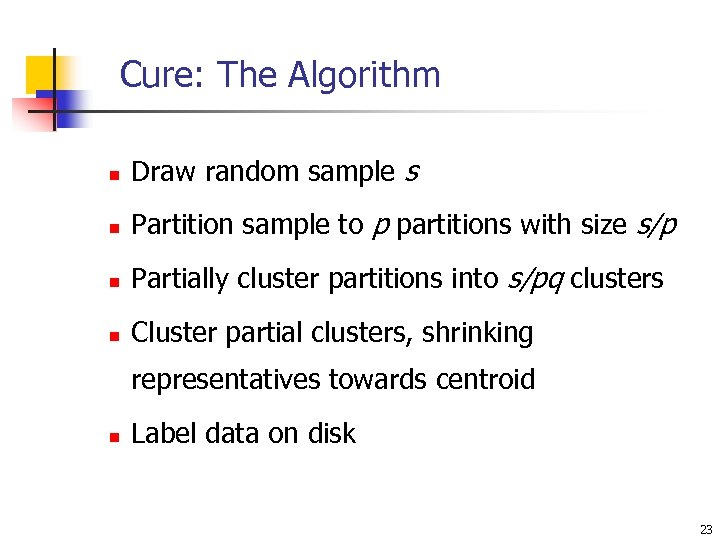Cure: The Algorithm n Draw random sample s n Partition sample to p partitions with size s/p n Partially cluster partitions into s/pq clusters n Cluster partial clusters, shrinking representatives towards centroid n Label data on disk 23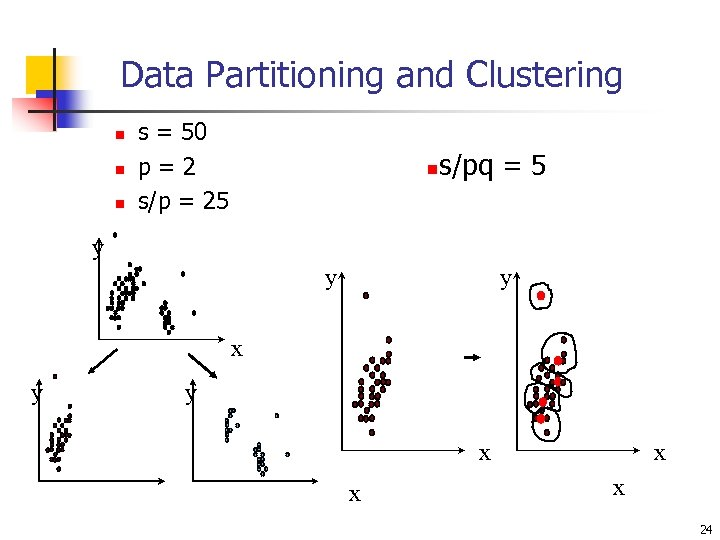Data Partitioning and Clustering n n n s = 50 p=2 s/p = 25 n s/pq = 5 y y y x x x x 24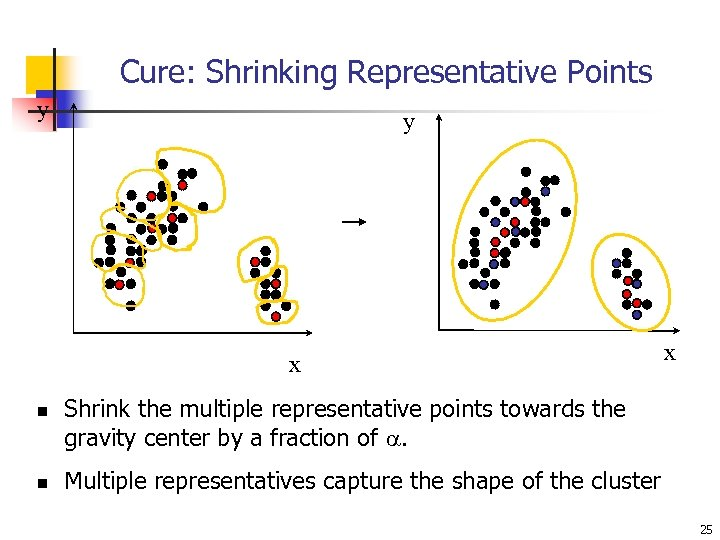Cure: Shrinking Representative Points y y x n n x Shrink the multiple representative points towards the gravity center by a fraction of . Multiple representatives capture the shape of the cluster 25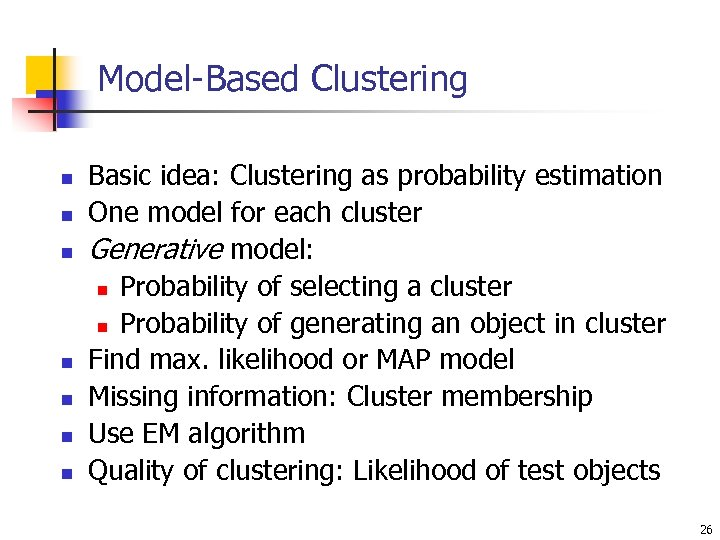Model-Based Clustering n n n n Basic idea: Clustering as probability estimation One model for each cluster Generative model: n Probability of selecting a cluster n Probability of generating an object in cluster Find max. likelihood or MAP model Missing information: Cluster membership Use EM algorithm Quality of clustering: Likelihood of test objects 26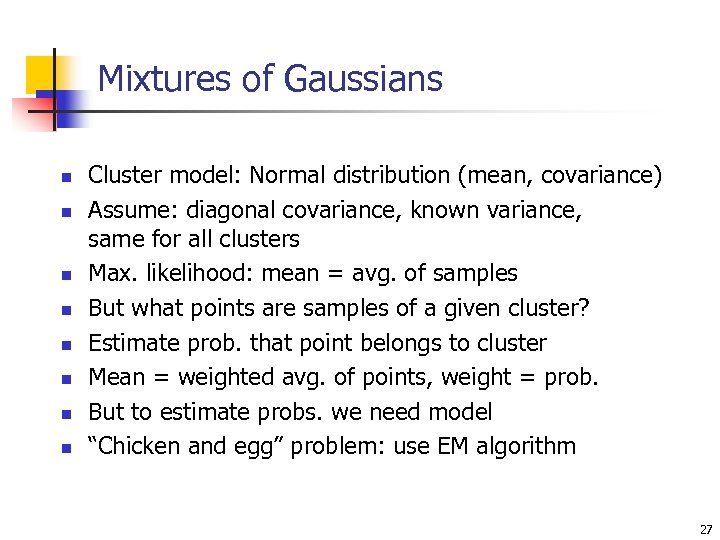Mixtures of Gaussians n n n n Cluster model: Normal distribution (mean, covariance) Assume: diagonal covariance, known variance, same for all clusters Max. likelihood: mean = avg. of samples But what points are samples of a given cluster? Estimate prob. that point belongs to cluster Mean = weighted avg. of points, weight = prob. But to estimate probs. we need model “Chicken and egg” problem: use EM algorithm 27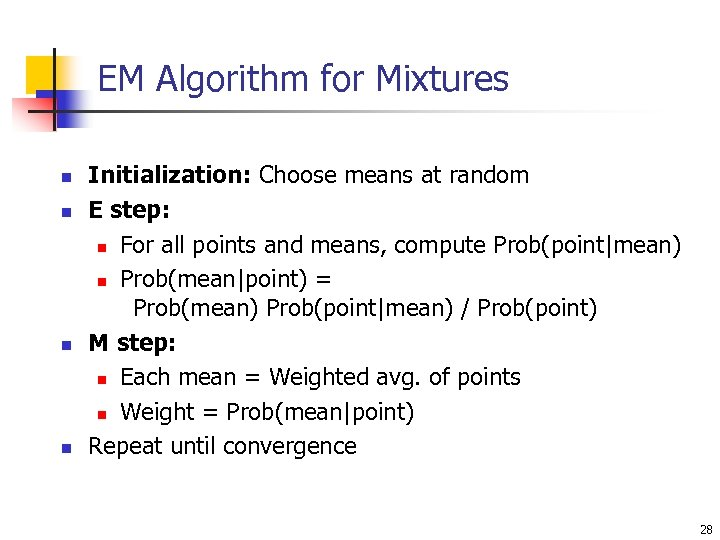EM Algorithm for Mixtures n n Initialization: Choose means at random E step: n For all points and means, compute Prob(point|mean) n Prob(mean|point) = Prob(mean) Prob(point|mean) / Prob(point) M step: n Each mean = Weighted avg. of points n Weight = Prob(mean|point) Repeat until convergence 28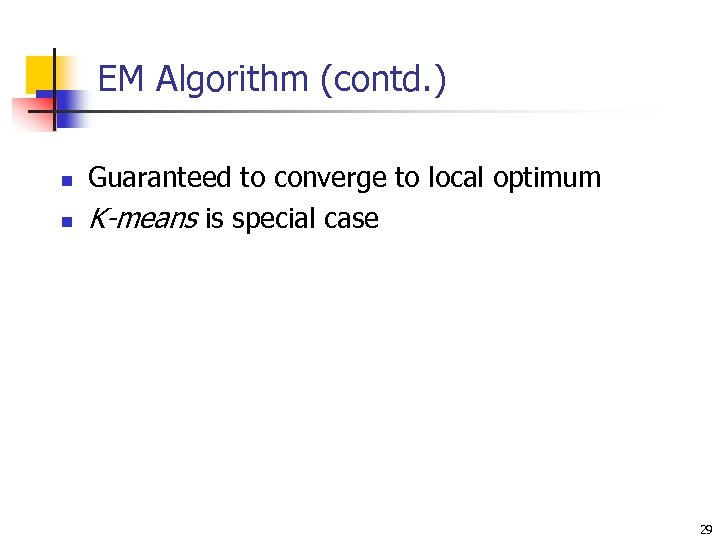EM Algorithm (contd. ) n n Guaranteed to converge to local optimum K-means is special case 29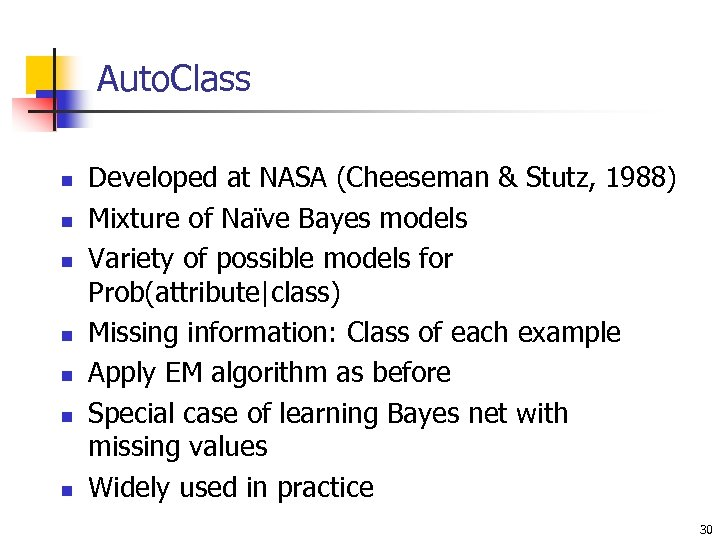Auto. Class n n n n Developed at NASA (Cheeseman & Stutz, 1988) Mixture of Naïve Bayes models Variety of possible models for Prob(attribute|class) Missing information: Class of each example Apply EM algorithm as before Special case of learning Bayes net with missing values Widely used in practice 30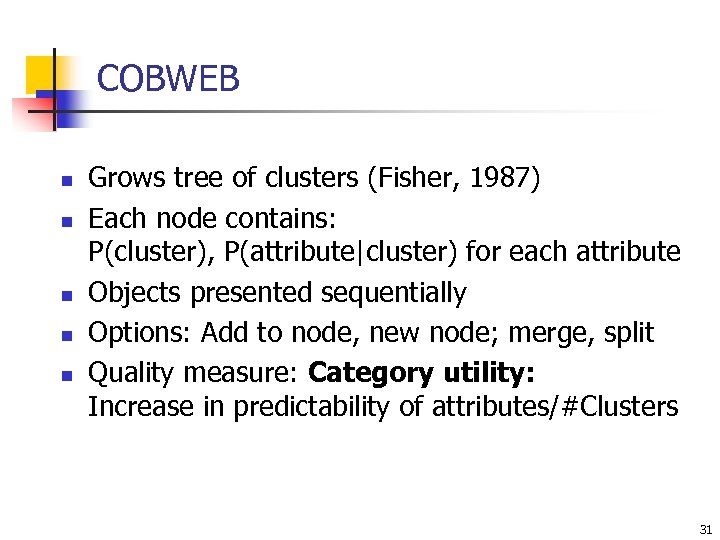COBWEB n n n Grows tree of clusters (Fisher, 1987) Each node contains: P(cluster), P(attribute|cluster) for each attribute Objects presented sequentially Options: Add to node, new node; merge, split Quality measure: Category utility: Increase in predictability of attributes/#Clusters 31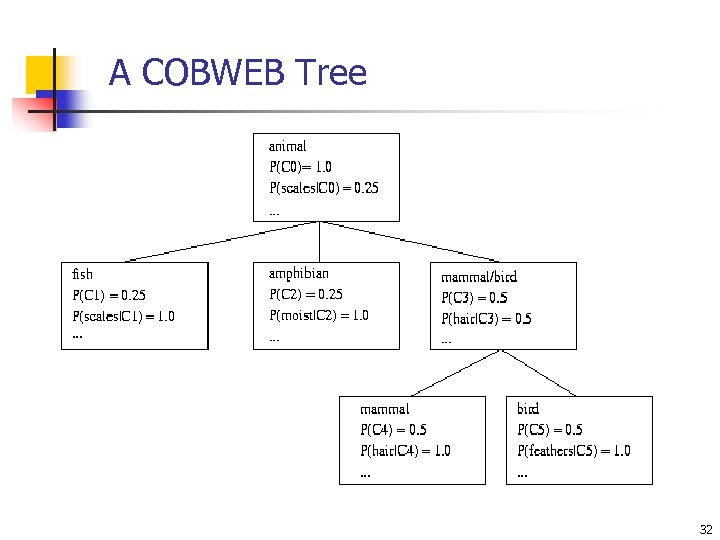A COBWEB Tree 32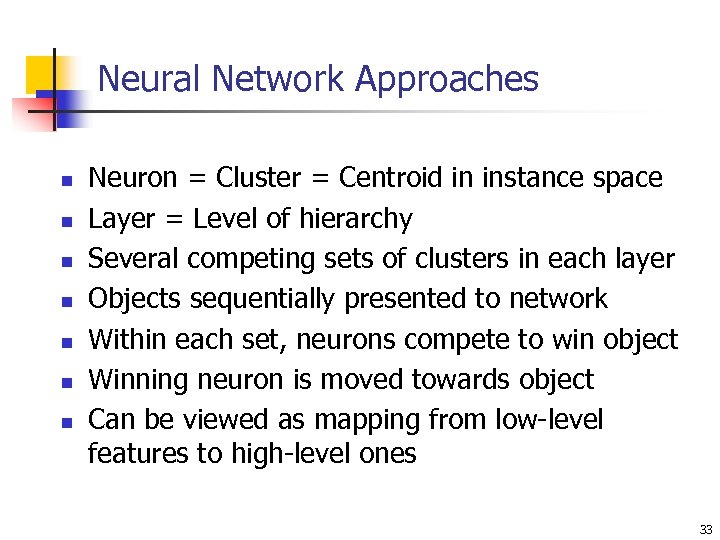Neural Network Approaches n n n n Neuron = Cluster = Centroid in instance space Layer = Level of hierarchy Several competing sets of clusters in each layer Objects sequentially presented to network Within each set, neurons compete to win object Winning neuron is moved towards object Can be viewed as mapping from low-level features to high-level ones 33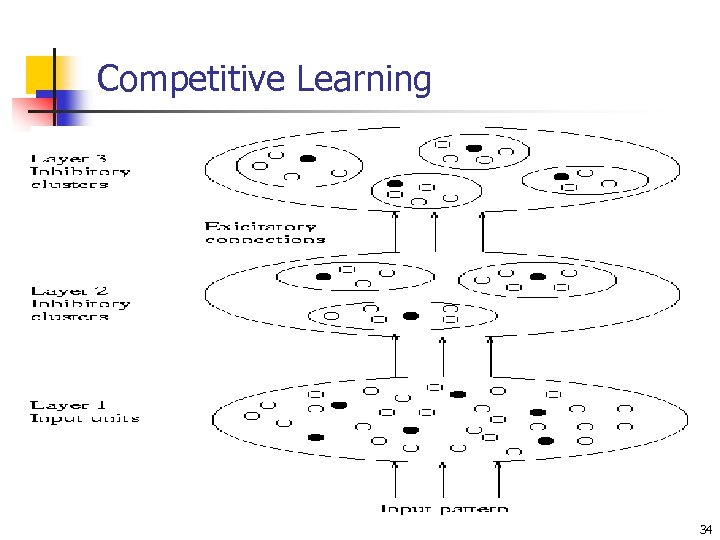Competitive Learning 34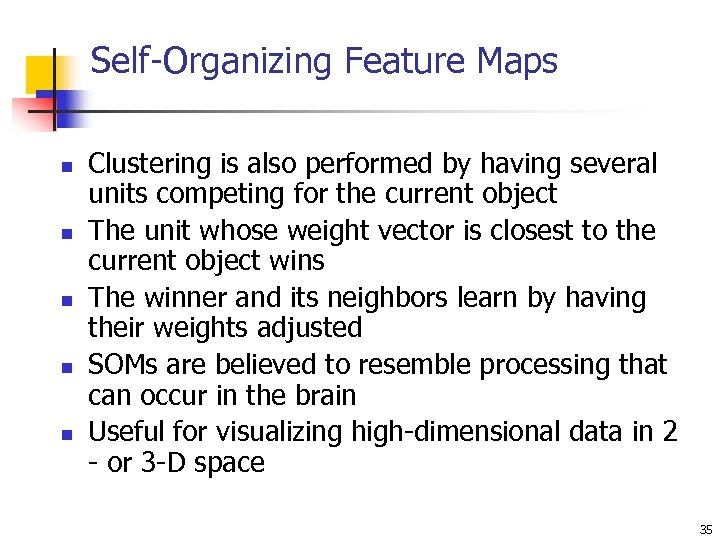Self-Organizing Feature Maps n n n Clustering is also performed by having several units competing for the current object The unit whose weight vector is closest to the current object wins The winner and its neighbors learn by having their weights adjusted SOMs are believed to resemble processing that can occur in the brain Useful for visualizing high-dimensional data in 2 - or 3 -D space 35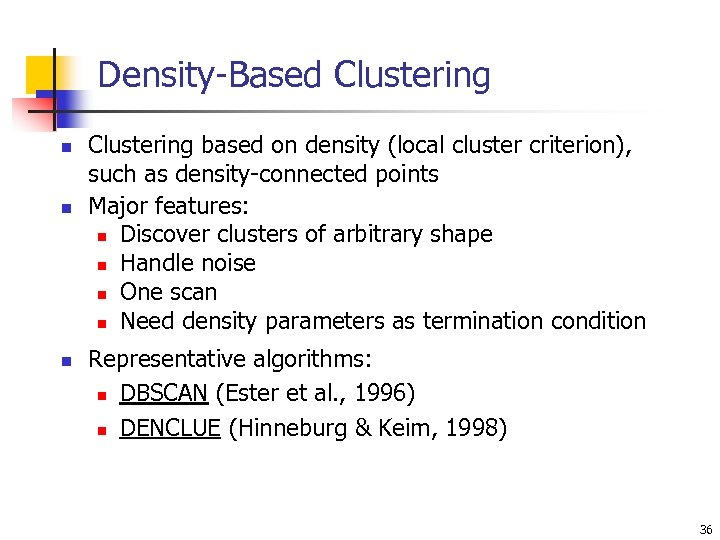Density-Based Clustering n n n Clustering based on density (local cluster criterion), such as density-connected points Major features: n Discover clusters of arbitrary shape n Handle noise n One scan n Need density parameters as termination condition Representative algorithms: n DBSCAN (Ester et al. , 1996) n DENCLUE (Hinneburg & Keim, 1998) 36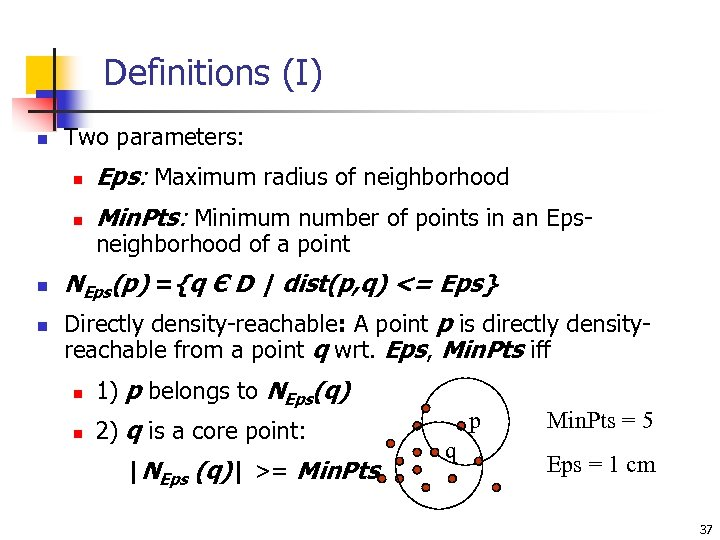Definitions (I) n Two parameters: n n Eps: Maximum radius of neighborhood Min. Pts: Minimum number of points in an Epsneighborhood of a point NEps(p) ={q Є D | dist(p, q) <= Eps} Directly density-reachable: A point p is directly densityreachable from a point q wrt. Eps, Min. Pts iff n 1) p belongs to NEps(q) n 2) q is a core point: |NEps (q)| >= Min. Pts p q Min. Pts = 5 Eps = 1 cm 37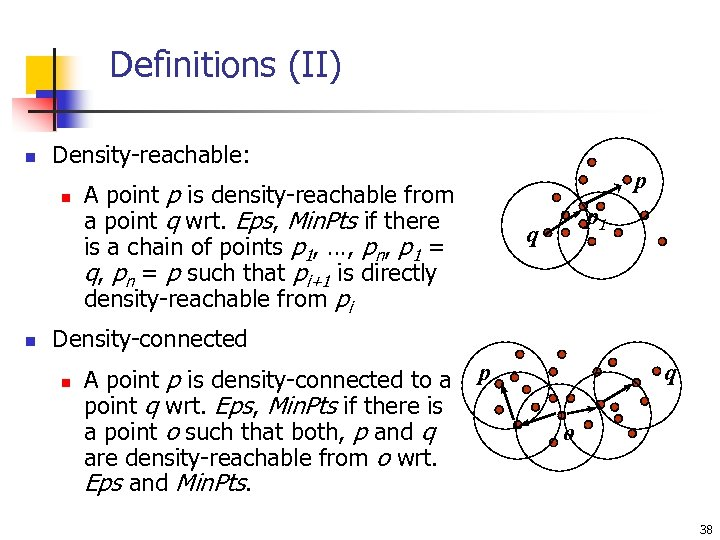Definitions (II) n Density-reachable: n n p A point p is density-reachable from a point q wrt. Eps, Min. Pts if there is a chain of points p 1, …, pn, p 1 = q, pn = p such that pi+1 is directly density-reachable from pi p 1 q Density-connected n A point p is density-connected to a point q wrt. Eps, Min. Pts if there is a point o such that both, p and q are density-reachable from o wrt. Eps and Min. Pts. p q o 38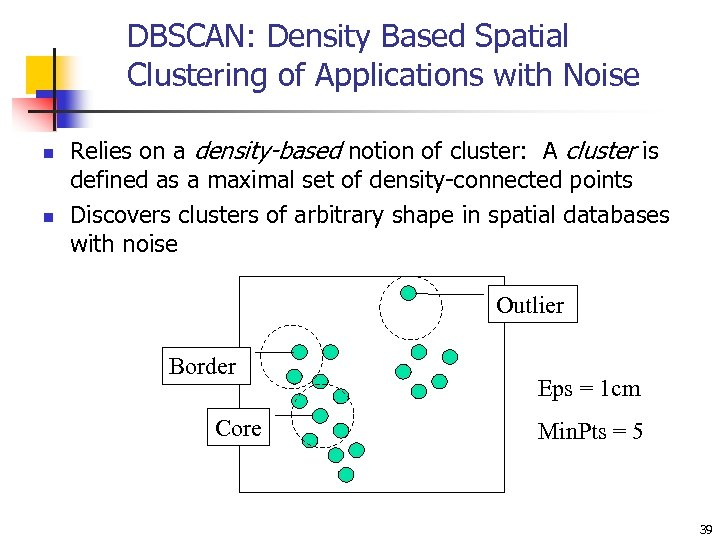DBSCAN: Density Based Spatial Clustering of Applications with Noise n n Relies on a density-based notion of cluster: A cluster is defined as a maximal set of density-connected points Discovers clusters of arbitrary shape in spatial databases with noise Outlier Border Core Eps = 1 cm Min. Pts = 5 39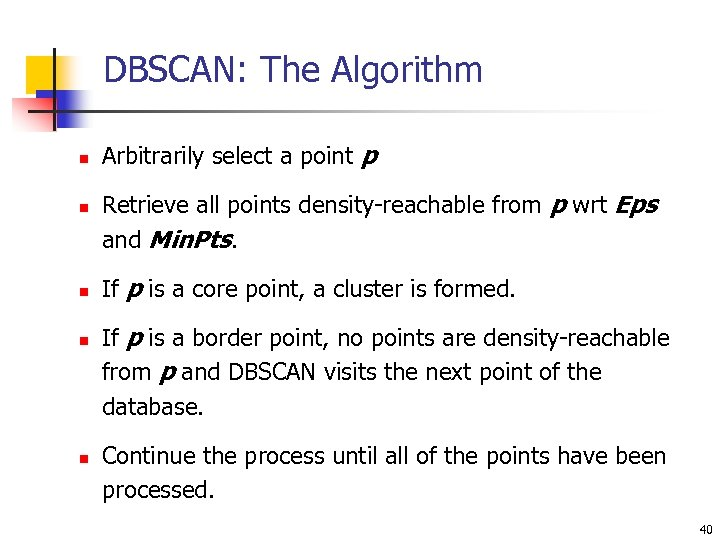DBSCAN: The Algorithm n n n Arbitrarily select a point p Retrieve all points density-reachable from p wrt Eps and Min. Pts. If p is a core point, a cluster is formed. If p is a border point, no points are density-reachable from p and DBSCAN visits the next point of the database. Continue the process until all of the points have been processed. 40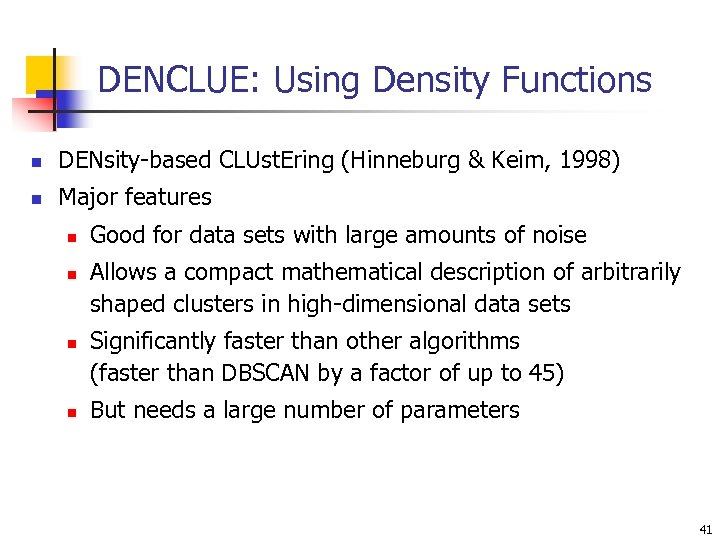DENCLUE: Using Density Functions n DENsity-based CLUst. Ering (Hinneburg & Keim, 1998) n Major features n n Good for data sets with large amounts of noise Allows a compact mathematical description of arbitrarily shaped clusters in high-dimensional data sets Significantly faster than other algorithms (faster than DBSCAN by a factor of up to 45) But needs a large number of parameters 41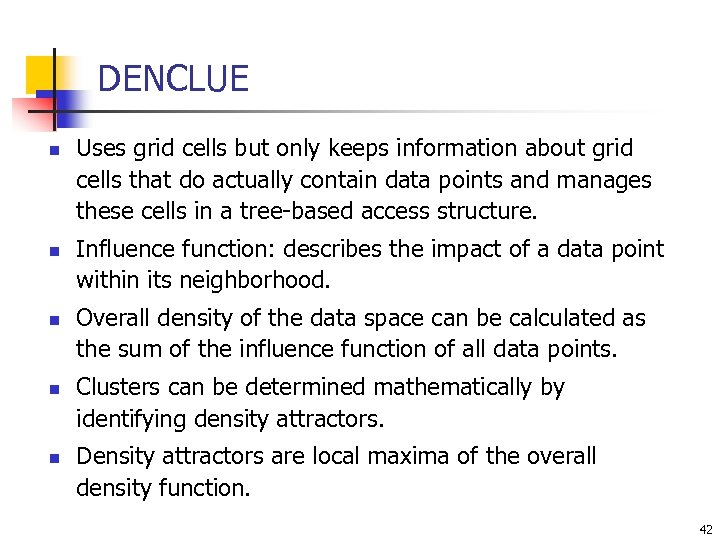DENCLUE n n n Uses grid cells but only keeps information about grid cells that do actually contain data points and manages these cells in a tree-based access structure. Influence function: describes the impact of a data point within its neighborhood. Overall density of the data space can be calculated as the sum of the influence function of all data points. Clusters can be determined mathematically by identifying density attractors. Density attractors are local maxima of the overall density function. 42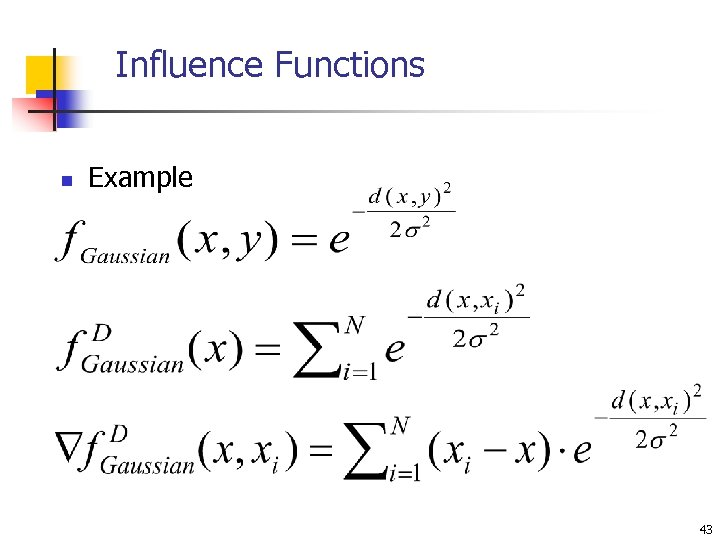Influence Functions n Example 43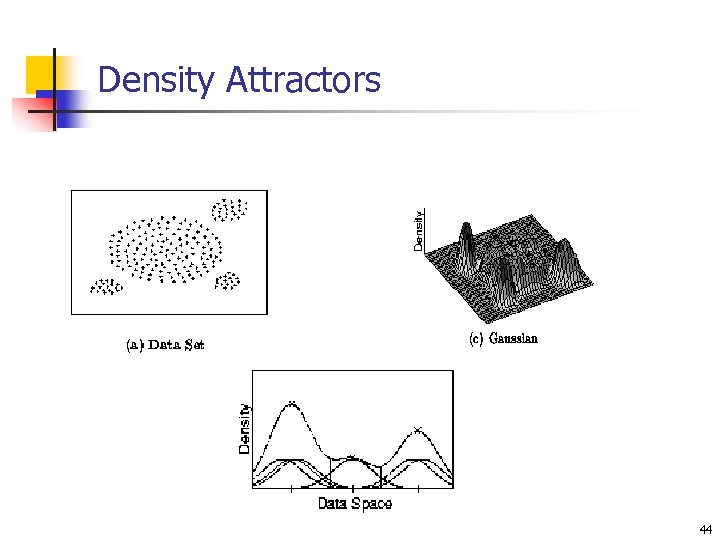Density Attractors 44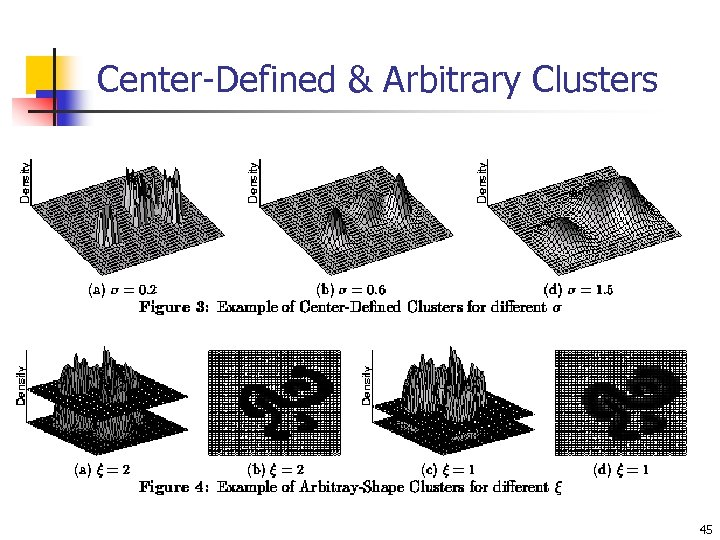Center-Defined & Arbitrary Clusters 45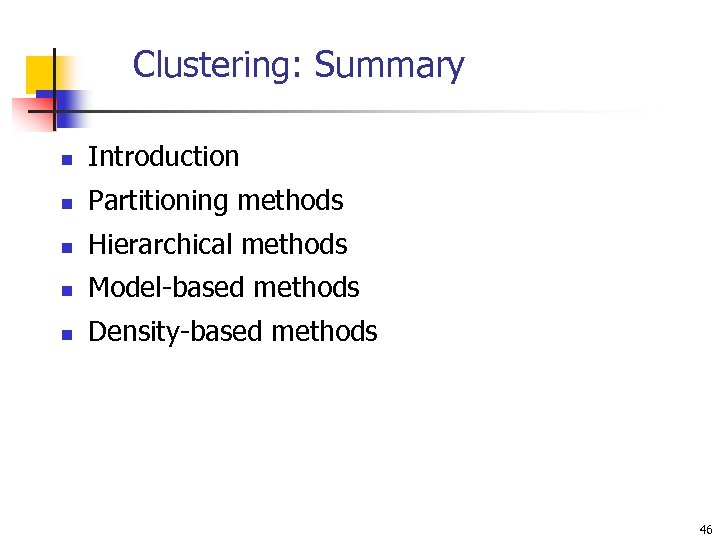Clustering: Summary n Introduction n Partitioning methods n Hierarchical methods n Model-based methods n Density-based methods 46